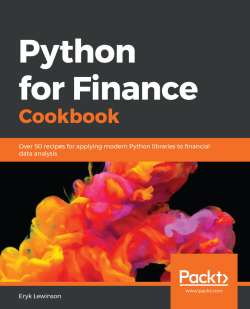### Python for Finance Cookbook5 (2 reviews total)
By Eryk Lewinson
• Constantly updated with 100+ new titles each month
• Breadth and depth in over 1,000+ technologies

Python is one of the most popular programming languages used in the financial industry, with a huge set of accompanying libraries.

In this book, you'll cover different ways of downloading financial data and preparing it for modeling. You'll calculate popular indicators used in technical analysis, such as Bollinger Bands, MACD, RSI, and backtest automatic trading strategies. Next, you'll cover time series analysis and models, such as exponential smoothing, ARIMA, and GARCH (including multivariate specifications), before exploring the popular CAPM and the Fama-French three-factor model. You'll then discover how to optimize asset allocation and use Monte Carlo simulations for tasks such as calculating the price of American options and estimating the Value at Risk (VaR). In later chapters, you'll work through an entire data science project in the financial domain. You'll also learn how to solve the credit card fraud and default problems using advanced classifiers such as random forest, XGBoost, LightGBM, and stacked models. You'll then be able to tune the hyperparameters of the models and handle class imbalance. Finally, you'll focus on learning how to use deep learning (PyTorch) for approaching financial tasks.

By the end of this book, you’ll have learned how to effectively analyze financial data using a recipe-based approach.

Publication date:
January 2020
Publisher
Packt
Pages
432
ISBN
9781789618518

# Financial Data and Preprocessing

The first chapter of this book is dedicated to a very important (if not the most important) part of any data science/quantitative finance project—gathering and working with data. In line with the "garbage in, garbage out" maxim, we should strive to have data of the highest possible quality, and correctly preprocess it for later use with statistical and machine learning algorithms. The reason for this is simple—the results of our analyses highly depend on the input data, and no sophisticated model will be able to compensate for that.

In this chapter, we cover the entire process of gathering financial data and preprocessing it into the form that is most commonly used in real-life projects. We begin by presenting a few possible sources of high-quality data, show how to convert prices into returns (which have properties desired by statistical algorithms), and investigate how to rescale asset returns (for example, from daily to monthly or yearly). Lastly, we learn how to investigate whether our data follows certain patterns (called stylized facts) commonly observed in financial assets.

One thing to bear in mind while reading this chapter is that data differs among sources, so the prices we see, for example, at Yahoo Finance and Quandl will most likely differ, as the respective sites also get their data from different sources and might use other methods to adjust the prices for corporate actions. The best practice is to find a source we trust the most concerning a particular type of data (based on, for example, opinion on the internet) and then use it for downloading data.

In this chapter, we cover the following recipes:

• Getting data from Yahoo Finance
• Getting data from Quandl
• Getting data from Intrinio
• Converting prices to returns
• Changing frequency
• Visualizing time series data
• Identifying outliers
• Investigating stylized facts of asset returns
The content presented in the book is valid for educational purposes only—we show how to apply different statistical/data science techniques to problems in the financial domain, such as stock price prediction and asset allocation. By no means should the information in the book be considered investment advice. Financial markets are very volatile and you should invest only at your own risk!

# Getting data from Yahoo Finance

One of the most popular sources of free financial data is Yahoo Finance. It contains not only historical and current stock prices in different frequencies (daily, weekly, monthly), but also calculated metrics, such as the beta (a measure of the volatility of an individual asset in comparison to the volatility of the entire market) and many more. In this recipe, we focus on retrieving historical stock prices.

For a long period of time, the go-to tool for downloading data from Yahoo Finance was the pandas-datareader library. The goal of the library was to extract data from a variety of sources and store it in the form of a pandas DataFrame. However, after some changes to the Yahoo Finance API, this functionality was deprecated. It is still good to be familiar with this library, as it facilitates downloading data from sources such as FRED (Federal Reserve Economic Data), the Fama/French Data Library or the World Bank, which might come in handy for different kinds of analyses (some of them are presented in the following chapters).

As of now, the easiest and fastest way of downloading historical stock prices is to use the yfinance library (formerly known as fix_yahoo_finance), which can be used on top of pandas-datareader or as a standalone library for downloading stock prices from Yahoo Finance. We focus on the latter use case.

For the sake of this example, we are interested in Apple's stock prices from the years 2000-2010.

# How to do it...

1. Import the libraries:
import pandas as pd import yfinance as yf
df_yahoo = yf.download('AAPL',                        start='2000-01-01',                        end='2010-12-31',                       progress=False)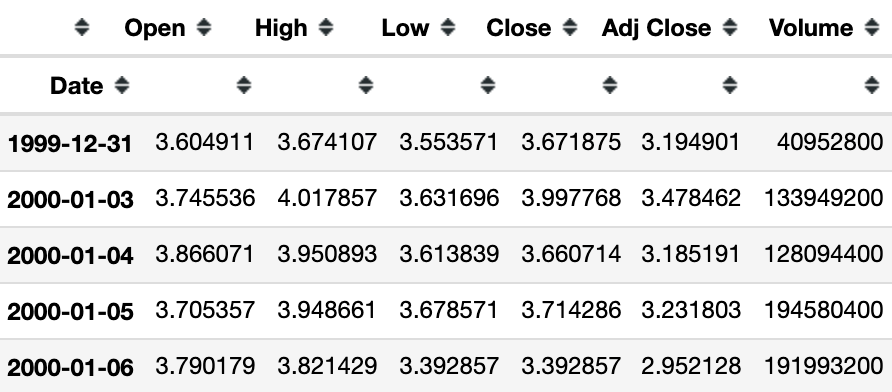The result of the request is a DataFrame (2,767 rows) containing daily Open, High, Low, and Close (OHLC) prices, as well as the adjusted close price and volume.

# How it works...

The download function is very intuitive; in the most basic case, we just need to provide the ticker (symbol), and it will try to download all data since 1950.

In the preceding example, we downloaded data from a specific range (2000-2010).

# There's more...

• We can pass a list of multiple tickers, such as ['AAPL', 'MSFT'].
• Setting progress=False disables the progress bar.

# Getting data from Quandl

Quandl is a provider of alternative data products for investment professionals, and offers an easy way to download data, also via a Python library.

A good starting place for financial data would be the WIKI Prices database, which contains stock prices, dividends, and splits for 3,000 US publicly traded companies. The drawback of this database is that as of April, 2018, it is no longer supported (meaning there is no recent data). However, for purposes of getting historical data or learning how to access the databases, it is more than enough.

We use the same example that we used in the previous recipe – we download Apple's stock prices for the years 2000-2010.

# How to do it...

1. Import the libraries:
import pandas as pd import quandl
1. Authenticate using the personal API key:
QUANDL_KEY = '{key}'quandl.ApiConfig.api_key = QUANDL_KEY

You need to replace {key} with your own API key.

df_quandl = quandl.get(dataset='WIKI/AAPL',                       start_date='2000-01-01',                        end_date='2010-12-31')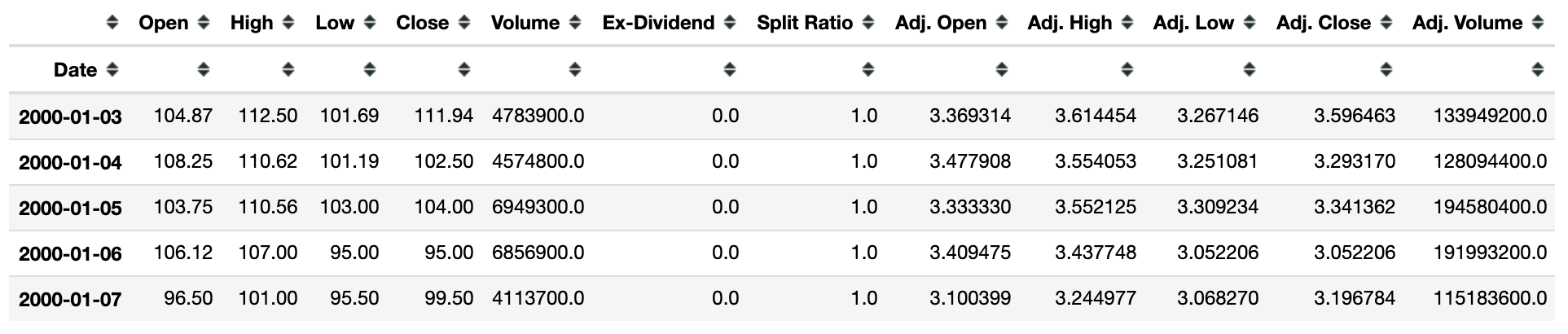The result of the request is a DataFrame (2,767 rows) containing the daily OHLC prices, the adjusted prices, dividends, and potential stock splits.

# How it works...

The first step after importing the required libraries was authentication using the API key (paste it instead of {key}). When providing the dataset argument, we used the following structure: DATASET/TICKER.

# There's more...

Some additional features of the get function are:

• We can specify multiple datasets at once using a list such as ['WIKI/AAPL', 'WIKI/MSFT'].
• The collapse parameter can be used to define the frequency (available options: daily, weekly, monthly, quarterly, or annually).

# Getting data from Intrinio

Another source of financial data is Intrinio, which offers access to its free (with limits) database. An additional feature is that we can also download already calculated technical indicators such as the Moving Average Convergence Divergence (MACD) and many more.

The database is not only restricted to stock prices, but we follow with the preceding example of downloading Apple's stock prices for the years 2000-2010.

# How to do it...

1. Import the libraries:
import intrinio_sdkimport pandas as pd
1. Authenticate using the personal API key, and select the API:
intrinio_sdk.ApiClient().configuration.api_key['api_key'] = '{key}' security_api = intrinio_sdk.SecurityApi()

You need to replace {key} with your own API key.

1. Request the data:
r = security_api.get_security_stock_prices(identifier='AAPL',                                            start_date='2000-01-01',                                           end_date='2010-12-31',                                            frequency='daily',                                                        page_size=10000)
1. Convert the results into a DataFrame:
response_list = [x.to_dict() for x in r.stock_prices]df_intrinio = pd.DataFrame(response_list).sort_values('date')df_intrinio.set_index('date', inplace=True)

The output looks like this: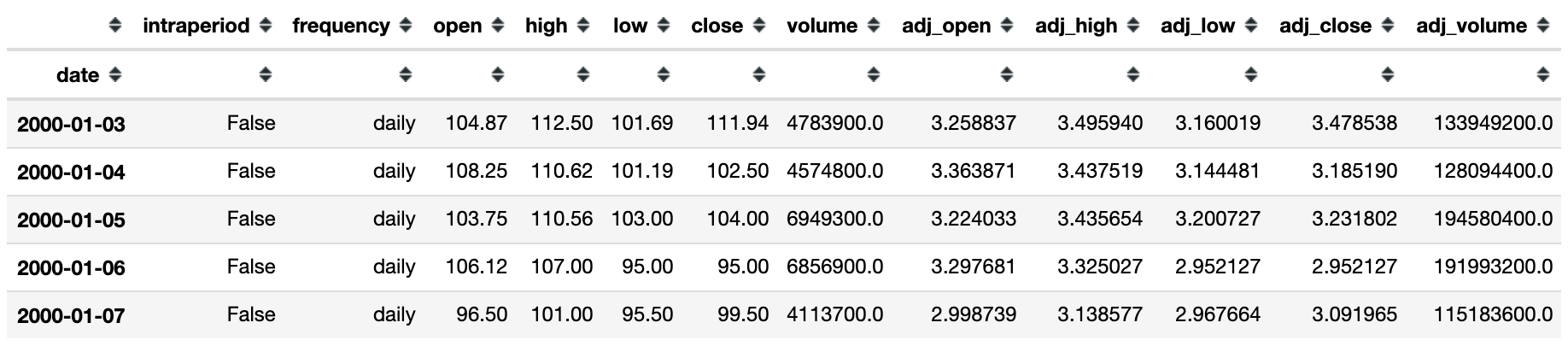The resulting DataFrame (2,771 rows) contains the OHLC prices and volume, as well as their adjusted counterparts.

# How it works...

The first step after importing the required libraries was to authenticate using the API key (paste it instead of {key}). Then, we selected the API we wanted to use for the recipe – in the case of stock prices, it was SecurityApi.

To download the data, we used the get_security_stock_prices method of the SecurityApi class. The parameters we can specify are as follows:

• identifier: Stock ticker or another acceptable identifier.
• start_date/end_date: This is self-explanatory.
• frequency: Which data frequency is of interest to us (available choices: daily, weekly, monthly, quarterly, or yearly).
• page_size: Defines the number of observations to return on one page; we set it to a high number to collect all data in one request with no need for the next_page token.

The API returns a JSON-like object, which we then transformed into a DataFrame and set the date as an index using the set_index method of a pandas DataFrame.

# There's more...

In this chapter, we have covered a few sources of financial data. Some additional, potentially interesting data sources are:

• iexfinance: A library that can be used to download data from IEX Cloud
• tiingo: A library that can be used to download data from Tiingo
• alpha_vantage: A library that is a wrapper for the Alpha Vantage API

# Converting prices to returns

Asset prices are usually non-stationary, that is, their statistics, such as mean and variance (mathematical moments) change over time. This could also mean observing some trends or seasonality in the price series (see Chapter 3, Time Series Modeling). By transforming the prices into returns, we attempt to make the time series stationary, which is the desired property in statistical modeling.

There are two types of returns:

• Simple returns: They aggregate over assets; the simple return of a portfolio is the weighted sum of the returns of the individual assets in the portfolio. Simple returns are defined as:• Log returns: They aggregate over time; it is easier to understand with the help of an examplethe log return for a given month is the sum of the log returns of the days within that month. Log returns are defined as:Pt is the price of an asset in time t. In the preceding case, we do not consider dividends, which obviously impact the returns and require a small modification of the formulas.

The best practice while working with stock prices is to use adjusted values, as they account for possible corporate actions, such as stock splits.

The difference between simple and log returns for daily/intraday data will be very small, however, the general rule is that log returns are smaller in value than simple returns.

In this recipe, we show how to calculate both types of returns using Apple's stock prices.

# How to do it...

Execute the following steps to download the stock prices and calculate simple/log returns.

1. Import the libraries:
import pandas as pd import numpy as npimport yfinance as yf
df = yf.download('AAPL',                  start='2000-01-01',                  end='2010-12-31',                 progress=False)df = df.loc[:, ['Adj Close']]df.rename(columns={'Adj Close':'adj_close'}, inplace=True)
1. Calculate the simple and log returns using the adjusted close prices:
df['simple_rtn'] = df.adj_close.pct_change()df['log_rtn'] = np.log(df.adj_close/df.adj_close.shift(1))

The resulting DataFrame looks as follows: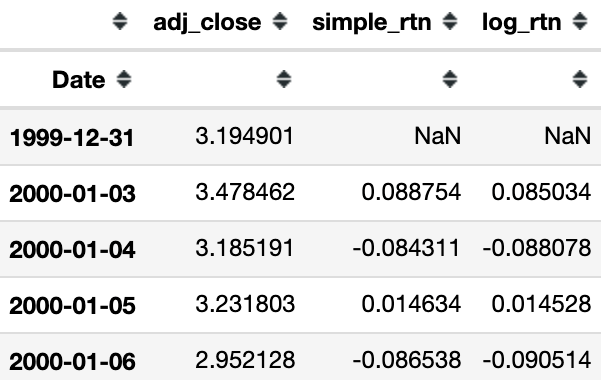The first row will always contain a not a number (NaN) value, as there is no previous price to use for calculating the returns.

# How it works...

In Step 2, we downloaded price data from Yahoo Finance, and only kept the adjusted close price for the returns calculation.

To calculate the simple returns, we used the pct_change method of pandas Series/DataFrame, which calculates the percentage change between the current and prior element (we can specify the number of lags, but for this specific case, the default value of 1 suffices).

To calculate the log returns, we followed the formula given in the introduction to this recipe. When dividing each element of the series by its lagged value, we used the shift method with a value of 1 to access the prior element. In the end, we took the natural logarithm of the divided values by using np.log.

# There's more...

We will also discuss how to account for inflation in the returns series. To do so, we continue with the example used in this recipe.

We first download the monthly Consumer Price Index (CPI) values from Quandl and calculate the percentage change (simple return) in the index. We can then merge the inflation data with Apple's stock returns, and account for inflation by using the following formula:Here, Rt is a time t simple return andis the inflation rate.

Execute the following steps to account for inflation in the returns series.

1. Import libraries and authenticate:
import pandas as pdimport quandlQUANDL_KEY = '{key}' quandl.ApiConfig.api_key = QUANDL_KEY

1. Create a DataFrame with all possible dates, and left join the prices to it:
df_all_dates = pd.DataFrame(index=pd.date_range(start='1999-12-31',                                                 end='2010-12-31'))df = df_all_dates.join(df[['adj_close']], how='left') \                 .fillna(method='ffill') \                 .asfreq('M')

We used a left join, which is a type of join (used for merging DataFrames) that returns all rows from the left table and the matched rows from the right table while leaving the unmatched rows empty. In case the last day of the month was not a trading day, we used the last known price of that month (fillna(method='ffill')). Lastly, we selected the end-of-month rows only by applying asfreq('M').

df_cpi = quandl.get(dataset='RATEINF/CPI_USA',                     start_date='1999-12-01',                     end_date='2010-12-31')df_cpi.rename(columns={'Value':'cpi'}, inplace=True)
1. Merge the inflation data to the prices:
df_merged = df.join(df_cpi, how='left')
1. Calculate the simple returns and inflation rate:
df_merged['simple_rtn'] = df_merged.adj_close.pct_change()df_merged['inflation_rate'] = df_merged.cpi.pct_change()
1. Adjust the returns for inflation:
df_merged['real_rtn'] = (df_merged.simple_rtn + 1) / (df_merged.inflation_rate + 1) - 1

The output looks as follows: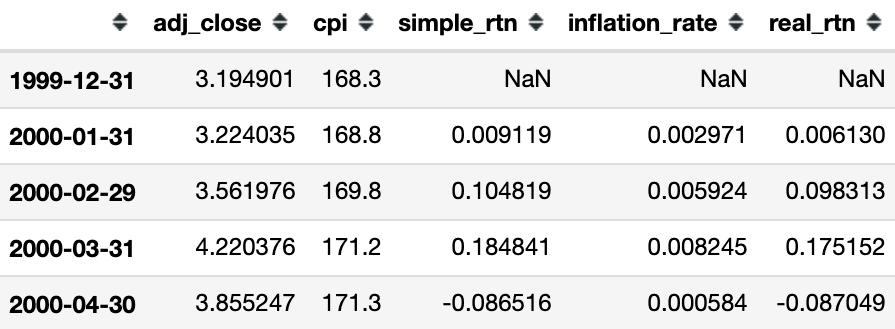The DataFrame contains all the intermediate results, and the real_rtn column contains the inflation-adjusted returns.

# Changing frequency

The general rule of thumb for changing frequency can be broken down into the following:

• Multiply/divide the log returns by the number of time periods.
• Multiply/divide the volatility by the square root of the number of time periods.

In this recipe, we present an example of how to calculate the monthly realized volatilities for Apple using daily returns and then annualize the values.

The formula for realized volatility is as follows:Realized volatility is frequently used for daily volatility using the intraday returns.

The steps we need to take are as follows:

• Calculate the realized volatility over the months.
• Annualize the values by multiplying by, as we are converting from monthly values.

We assume you have followed the instructions from earlier recipes and have a DataFrame called df with a single log_rtn column and timestamps as the index.

# How to do it...

Execute the following steps to calculate and annualize the monthly realized volatility.

1. Import the libraries:
import pandas as pd
1. Define the function for calculating the realized volatility:
def realized_volatility(x):    return np.sqrt(np.sum(x**2))
1. Calculate the monthly realized volatility:
df_rv = df.groupby(pd.Grouper(freq='M')).apply(realized_volatility)df_rv.rename(columns={'log_rtn': 'rv'}, inplace=True)
1. Annualize the values:
df_rv.rv = df_rv.rv * np.sqrt(12)
1. Plot the results:
fig, ax = plt.subplots(2, 1, sharex=True)ax.plot(df)ax.plot(df_rv)

Executing the preceding code results in the following plots: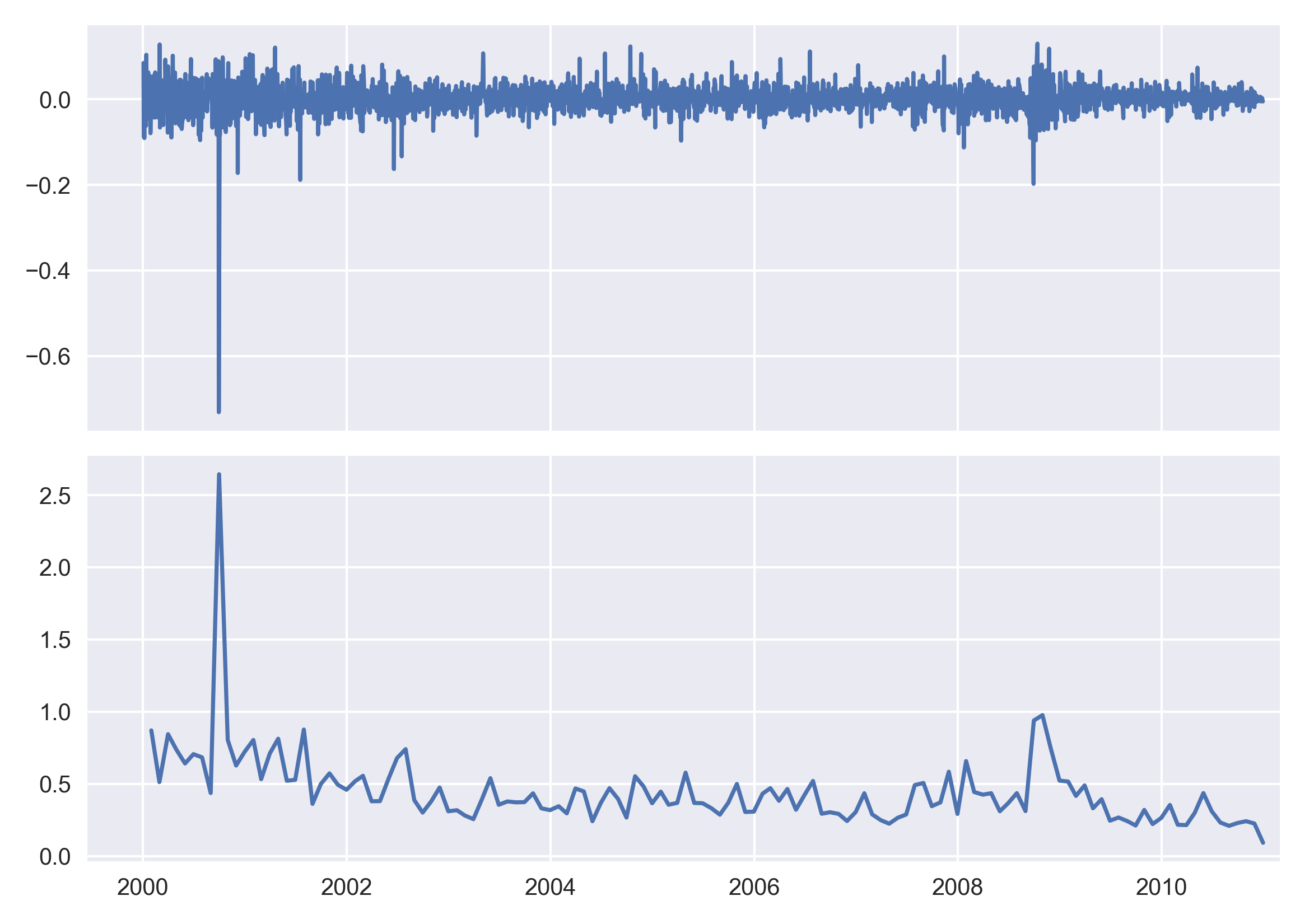We can see that the spikes in the realized volatility coincide with some extreme returns (which might be outliers).

# How it works...

Normally, we could use the resample method of a pandas DataFrame. Supposing we wanted to calculate the average monthly return, we could run df.log_rtn.resample('M').mean().

For the resample method, we can use any built-in aggregate functions of pandas, such as mean, sum, min, and max. However, our case is a bit more complex, so we defined a helper function called realized_volatility, and replicated the behavior of resample by using a combination of groupby, Grouper, and apply.

We presented the most basic visualization of the results (please refer to the next recipe for information about visualizing time series).

# Visualizing time series data

After learning how to download and preprocess financial data, it is time to learn how to plot it in a visually appealing way. We cover two approaches using the following:

• The default plot method of a pandas DataFrame
• A combination of the plotly and cufflinks libraries

The plotly library is built on top of d3.js (a JavaScript library used for creating interactive visualizations in web browsers) and is known for creating high-quality plots with a significant degree of interactivity (inspecting values of observations, viewing tooltips of a given point, zooming in, and so on). Plotly is also the company responsible for developing this library and provides hosting for our visualizations. We can create an infinite number of offline visualizations and up to 25 free ones to share online (with a limited number of views per day).

The cufflinks library also makes the process easier, as it enables us to create the plotly visualizations directly on top of pandas DataFrames.

In this recipe, we plot Microsoft's stock prices (all-time) and returns. For details on how to download and preprocess the data, please refer to the earlier recipes.

For this recipe, we assume we already have a DataFrame called df with three columns (adj_close, simple_rtn, and log_rtn) and dates set as the index. Please refer to the notebook on the GitHub repository for details on downloading data for this recipe.

# How to do it...

In this section, we introduce how to plot time series data. We start by using the default plot method of a pandas DataFrame/Series, and then present the interactive alternative offered by the combination of plotly and cufflinks.

# The plot method of pandas

Execute the following code to plot Microsoft's stock prices together with the simple and log returns.

fig, ax = plt.subplots(3, 1, figsize=(24, 20), sharex=True)df.adj_close.plot(ax=ax)ax.set(title = 'MSFT time series',          ylabel = 'Stock price ($)') df.simple_rtn.plot(ax=ax)ax.set(ylabel = 'Simple returns (%)')df.log_rtn.plot(ax=ax)ax.set(xlabel = 'Date', ylabel = 'Log returns (%)') Executing the preceding code results in the following plot: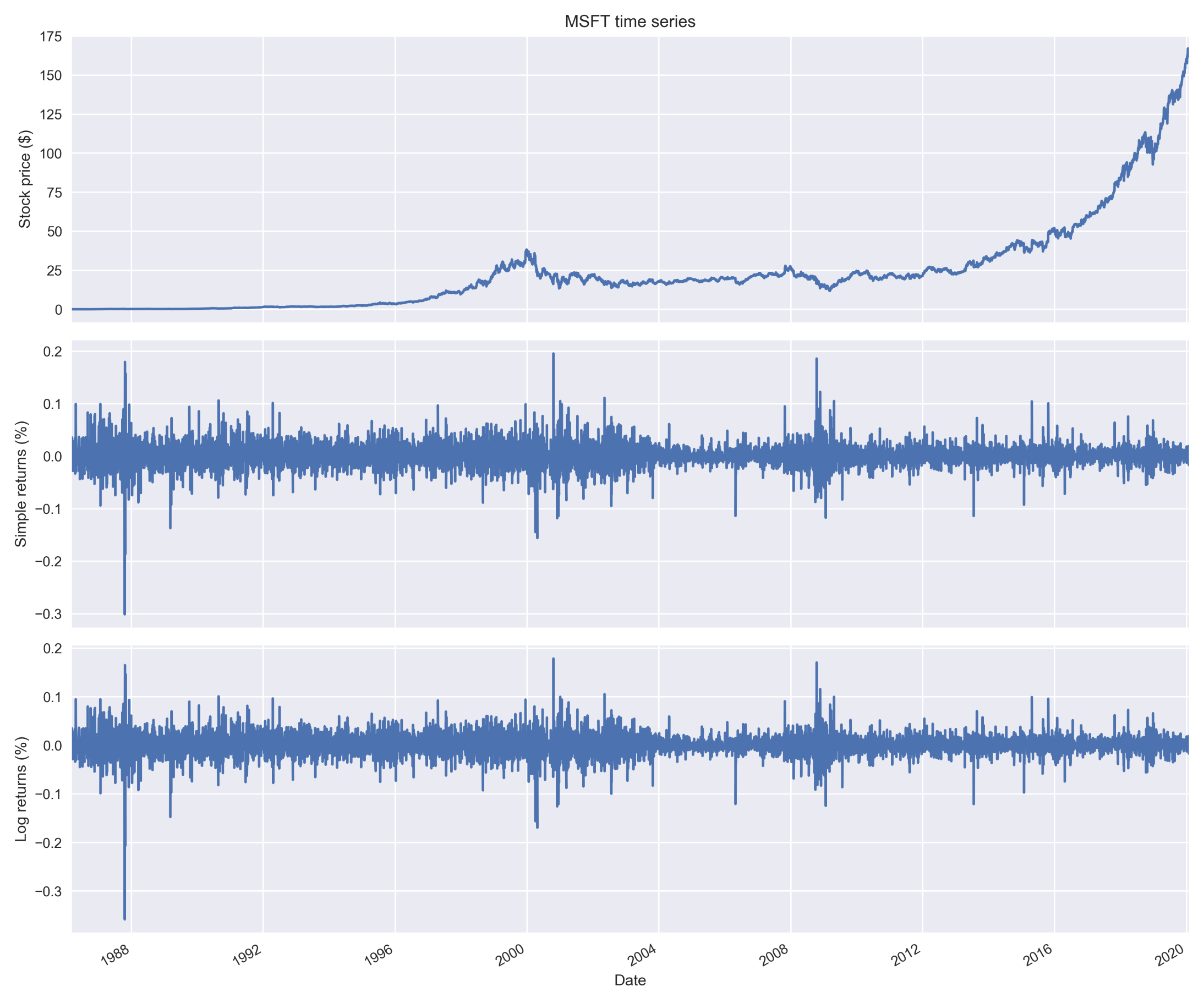The resulting plot contains three axes. Each one of them presents a different series: raw prices, simple returns, and log returns. Inspecting the plot in such a setting enables us to see the periods of heightened volatility and what was happening at the same time with the price of Microsoft's stock. Additionally, we see how similar simple and log returns are. # plotly and cufflinks Execute the following code to plot Microsoft's stock prices together with the simple and log returns. 1. Import the libraries and handle the settings: import cufflinks as cffrom plotly.offline import iplot, init_notebook_mode# set up configuration (run it once)#cf.set_config_file(world_readable=True, theme='pearl', # offline=True)init_notebook_mode() 1. Create the plot: df.iplot(subplots=True, shape=(3,1), shared_xaxes=True, title='MSFT time series') We can observe the time series in the following plot: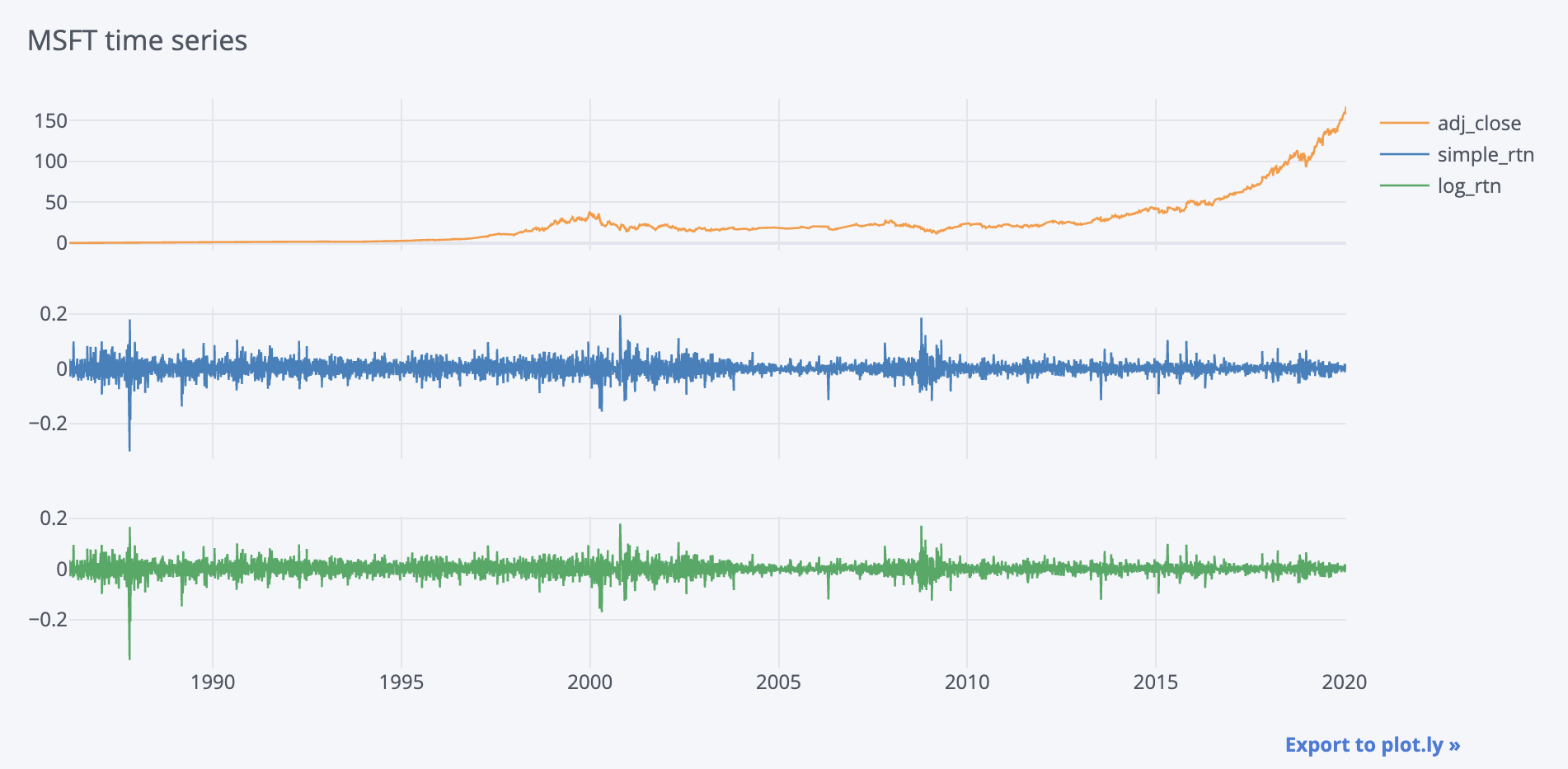The main advantage of using plotly with cufflinks is the interactivity of the preceding chart, which is unfortunately only demonstrable in the Notebook (please refer to the accompanying GitHub repository). # How it works... In the next section, we go over the details of the two selected methods of plotting time series in Python. # The plot method of pandas Our goal was to visualize all three series on the same plot (sharing the x-axis) to enable a quick visual comparison. To achieve this, we had to complete the following steps: • We created a subplot, which we then populated with individual plots. We specified that we wanted three plots vertically (by indicating plt.subplots(3, 1)). We also specified the figure size by setting the figsize parameter. • We added the individual plots using the plot method on a single Series (column) and specifying the axis on which we wanted to place the plot. • We used the set method to specify the title and axis labels on each of the plots. When working in Jupyter Notebook, best practice is to run the %matplotlib inline magic (once per kernel) in order to display the plots directly below the code cell that has produced it. Additionally, if you are working on a MacBook with a Retina screen, running the extra IPython magic %config InlineBackend.figure_format ='retina' will double the resolution of the plots we make. Definitely worth the extra line! # plotly and cufflinks By using cufflinks, we can use the iplot method directly on a pandas DataFrame. To create the previous plot, we used subplots (subplots=True), specified the shape of the figure (shape=(3,1)), indicated that the plots share the x-axis (shared_xaxes=True), and added the title (title='MSFT time series'). By default, the selected type of plot is a line chart (kind='line'). One note about using plotly in Jupyter—in order to share a notebook with the option to view the plot (without running the script again), you should use nbviewer or render the notebook as an HTML file and share it then. The extra line of code cf.set_config_file(world_readable=True, theme='pearl', offline=True) sets up the configuration (such as the current theme or the offline mode) and should be used only once. It can be used again to reconfigure. # There's more... There are many more ways to create plots in Python. We list some of the libraries: • matplotlib • seaborn • plotly • plotly_express • altair • plotnine We have decided to present the two selected for their simplicity, however, a specific use case might require using some of the previously mentioned libraries as they offer more freedom when creating the visualization. We should also mention that the plot method of a pandas DataFrame actually uses matplotlib for plotting, but the pandas API makes the process easier. # See also # Identifying outliers While working with any kind of data, we often encounter observations that are significantly different from the majority, that is, outliers. They can be a result of a wrong tick (price), something major happening on the financial markets, an error in the data processing pipeline, and so on. Many machine learning algorithms and statistical approaches can be influenced by outliers, leading to incorrect/biased results. That is why we should handle the outliers before creating any models. In this recipe, we look into detecting outliers using the 3σ approach. # Getting ready We continue from the Converting prices to returns recipe and have a DataFrame with Apple's stock price history and returns. # How to do it... Execute the following steps to detect outliers using the 3σ approach, and mark them on a plot. 1. Calculate the rolling mean and standard deviation: df_rolling = df[['simple_rtn']].rolling(window=21) \ .agg(['mean', 'std'])df_rolling.columns = df_rolling.columns.droplevel() 1. Join the rolling metrics to the original data: df_outliers = df.join(df_rolling) 1. Define a function for detecting outliers: def indentify_outliers(row, n_sigmas=3): x = row['simple_rtn'] mu = row['mean'] sigma = row['std'] if (x > mu + 3 * sigma) | (x < mu - 3 * sigma): return 1 else: return 0 1. Identify the outliers and extract their values for later use: df_outliers['outlier'] = df_outliers.apply(indentify_outliers, axis=1) outliers = df_outliers.loc[df_outliers['outlier'] == 1, ['simple_rtn']]  1. Plot the results: fig, ax = plt.subplots()ax.plot(df_outliers.index, df_outliers.simple_rtn, color='blue', label='Normal')ax.scatter(outliers.index, outliers.simple_rtn, color='red', label='Anomaly')ax.set_title("Apple's stock returns")ax.legend(loc='lower right') Executing the code results in the following plot: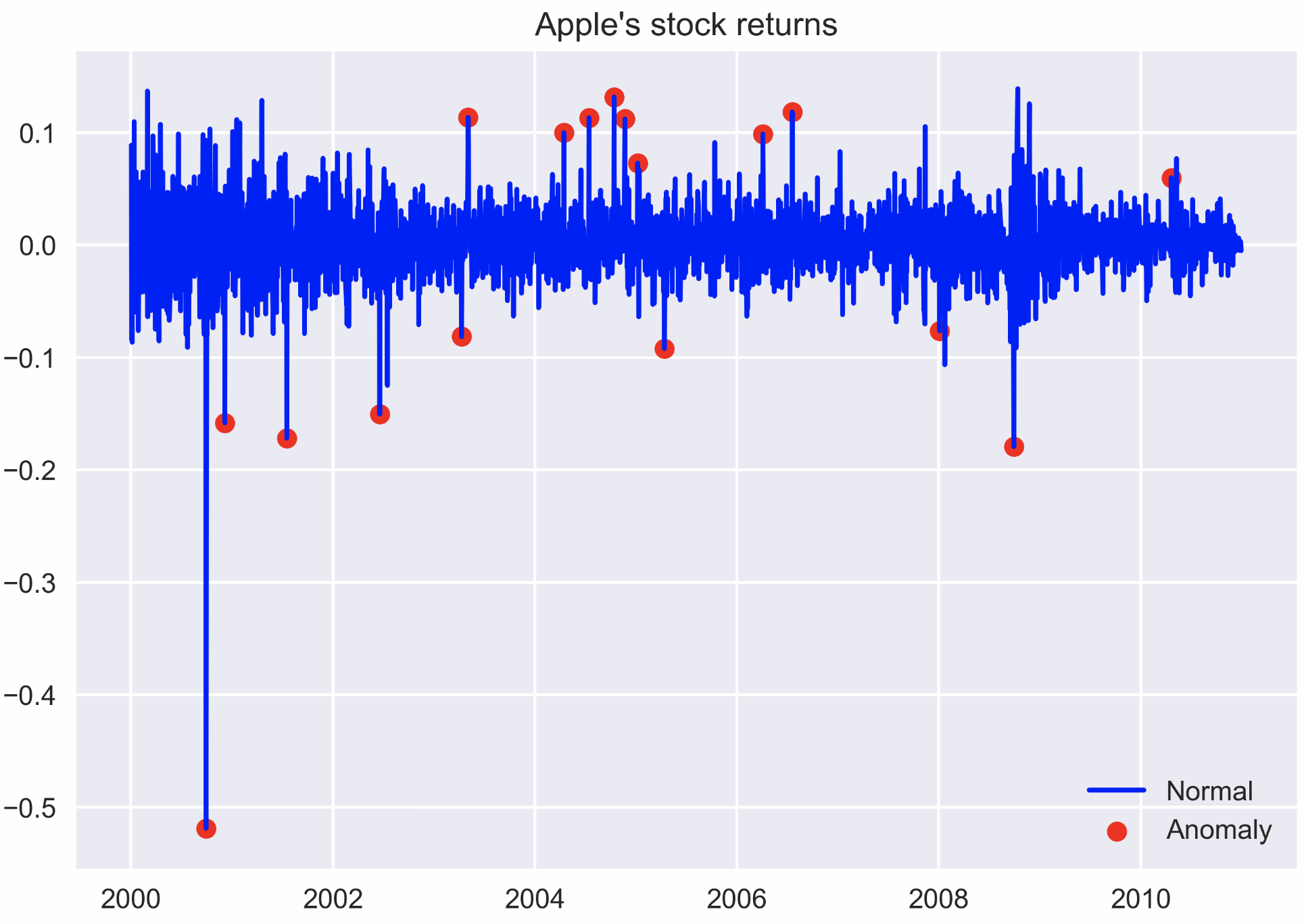In the plot, we can observe outliers marked with a red dot. One thing to notice is that when there are two large returns in the vicinity, the algorithm identifies the first one as an outlier and the second one as a regular observation. This might be due to the fact that the first outlier enters the rolling window and affects the moving average/standard deviation. We should also be aware of the so-called ghost effect/feature. When a single outlier enters the rolling window, it inflates the values of the rolling statistics for as long as it is in the window. # How it works... In the 3σ approach, for each time point, we calculated the moving average (μ) and standard deviation (σ) using the last 21 days (not including that day). We used 21 as this is the average number of trading days in a month, and we work with daily data. However, we can choose different values, and then the moving average will react faster/slower to changes. We can also use (exponentially) weighted moving average if we find it more meaningful in our particular case. The condition for a given observation x to be qualified as an outlier is x > μ + 3σ or x < μ - 3σ. In the first step, we calculated the rolling metrics using the rolling method of a pandas DataFrame. We specified the window's size and the metrics we would like to calculate. In the second step, we joined the two DataFrames. In Step 3, we defined a function that returns 1 if the observation is considered an outlier, according to the 3σ rule (we parametrized the number of standard deviations), and 0 otherwise. Then, in the fourth step, we applied the function to all rows in the DataFrame using the apply method. In the last step, we visualized the returns series and marked the outliers using a red dot. In real-life cases, we should not only identify the outliers, but also treat them, for example, by capping them at the maximum/minimum acceptable value, replacing them by interpolated values, or by following any of the other possible approaches. # There's more... There are many different methods of identifying outliers in a time series, for example, using Isolation Forest, Hampel Filter, Support Vector Machines, and z-score (which is similar to the presented approach). # Investigating stylized facts of asset returns Stylized facts are statistical properties that appear to be present in many empirical asset returns (across time and markets). It is important to be aware of them because when we are building models that are supposed to represent asset price dynamics, the models must be able to capture/replicate these properties. In the following recipes, we investigate the five stylized facts using an example of daily S&P 500 returns from the years 1985 to 2018. # Getting ready We download the S&P 500 prices from Yahoo Finance (following the approach in the Getting data from Yahoo Finance recipe) and calculate returns as in the Converting prices to returns recipe. We use the following code to import all the required libraries: import pandas as pd import numpy as npimport yfinance as yfimport seaborn as sns import scipy.stats as scsimport statsmodels.api as smimport statsmodels.tsa.api as smt # How to do it... In this section, we investigate, one by one, five stylized facts in the S&P 500 series. # Non-Gaussian distribution of returns Run the following steps to investigate the existence of this first fact by plotting the histogram of returns and a Q-Q plot. 1. Calculate the normal Probability Density Function (PDF) using the mean and standard deviation of the observed returns: r_range = np.linspace(min(df.log_rtn), max(df.log_rtn), num=1000)mu = df.log_rtn.mean()sigma = df.log_rtn.std()norm_pdf = scs.norm.pdf(r_range, loc=mu, scale=sigma)  1. Plot the histogram and the Q-Q plot: fig, ax = plt.subplots(1, 2, figsize=(16, 8))# histogramsns.distplot(df.log_rtn, kde=False, norm_hist=True, ax=ax) ax.set_title('Distribution of MSFT returns', fontsize=16) ax.plot(r_range, norm_pdf, 'g', lw=2, label=f'N({mu:.2f}, {sigma**2:.4f})')ax.legend(loc='upper left');# Q-Q plotqq = sm.qqplot(df.log_rtn.values, line='s', ax=ax)ax.set_title('Q-Q plot', fontsize = 16) Executing the preceding code results in the following plot: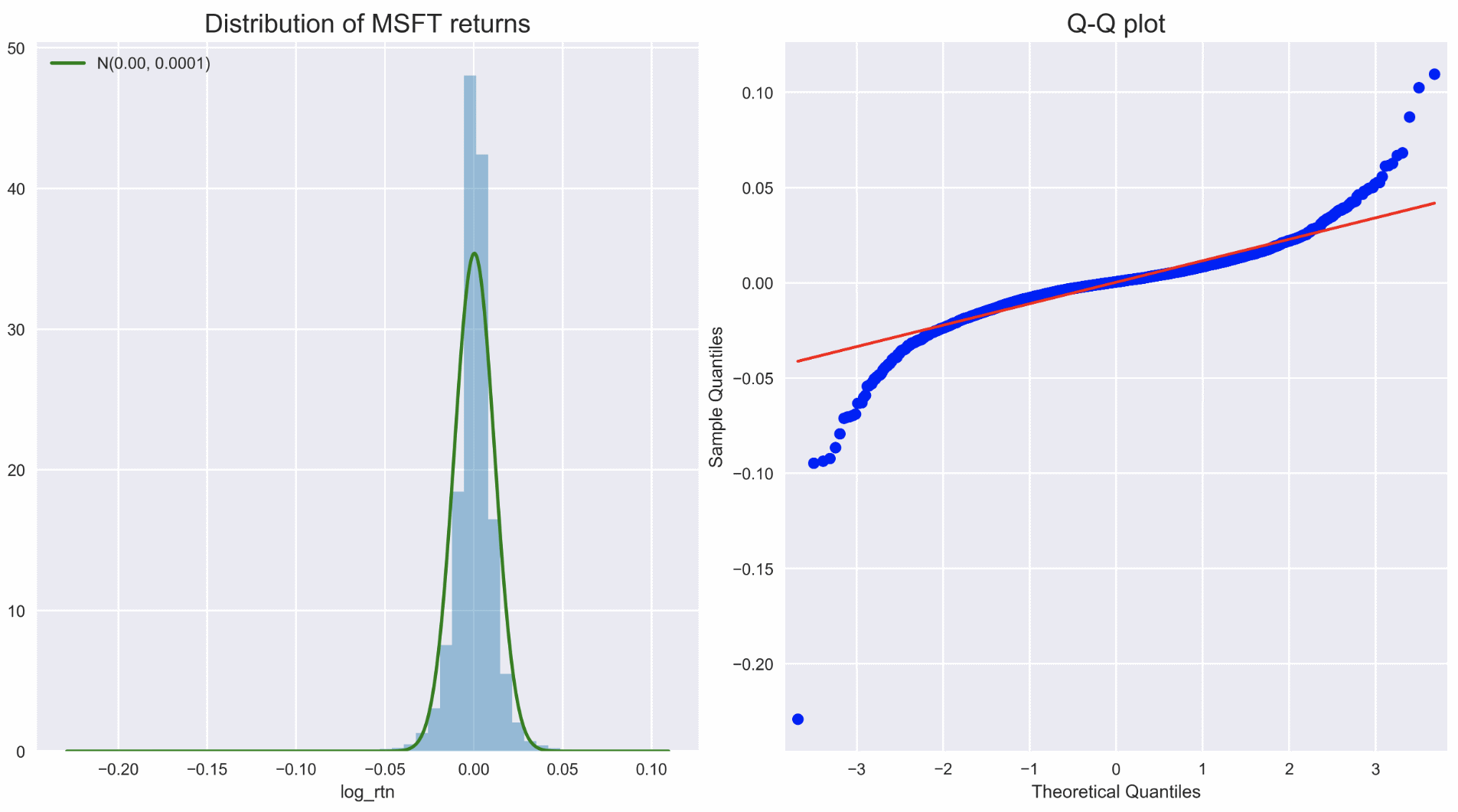We can use the histogram (showing the shape of the distribution) and the Q-Q plot to assess the normality of the log returns. Additionally, we can print the summary statistics (please refer to the GitHub repository for the code):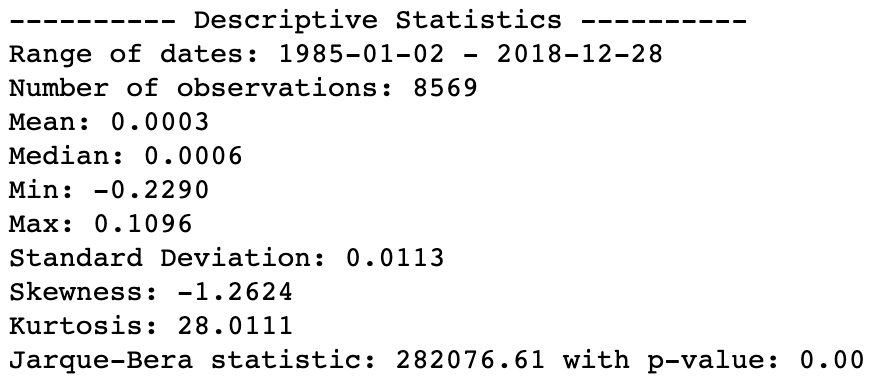By looking at the metrics such as the mean, standard deviation, skewness, and kurtosis we can infer that they deviate from what we would expect under normality. Additionally, the Jarque-Bera normality test gives us reason to reject the null hypothesis stating that the distribution is normal at the 99% confidence level. # Volatility clustering Run the following code to investigate this second fact by plotting the log returns series. 1. Visualize the log returns series: df.log_rtn.plot(title='Daily MSFT returns') Executing the code results in the following plot: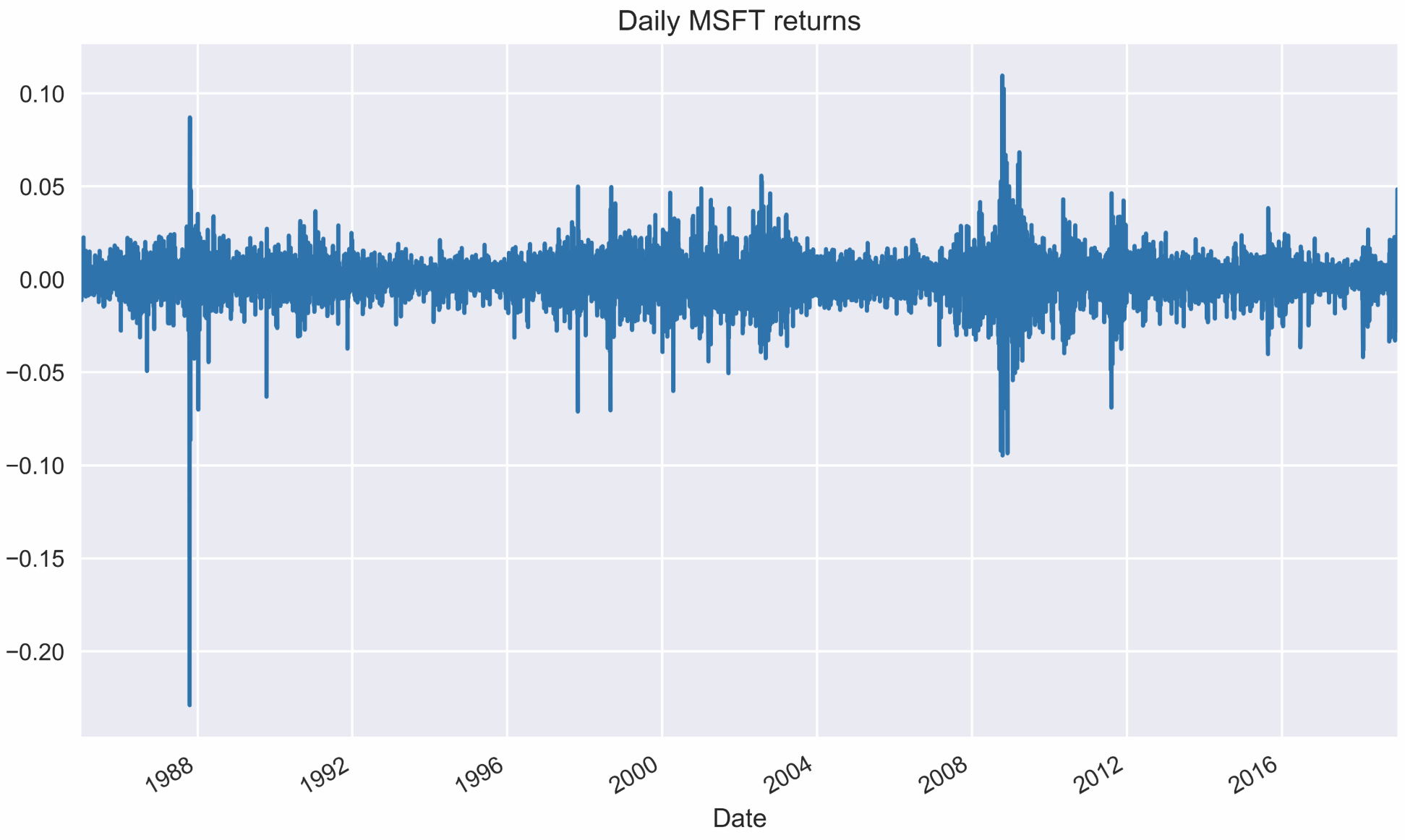We can observe clear clusters of volatility—periods of higher positive and negative returns. # Absence of autocorrelation in returns Investigate this third fact about the absence of autocorrelation in returns. 1. Define the parameters for creating the autocorrelation plots: N_LAGS = 50SIGNIFICANCE_LEVEL = 0.05 1. Run the following code to create the autocorrelation function (ACF) plot of log returns: acf = smt.graphics.plot_acf(df.log_rtn, lags=N_LAGS, alpha=SIGNIFICANCE_LEVEL) Executing the preceding code results in the following plot: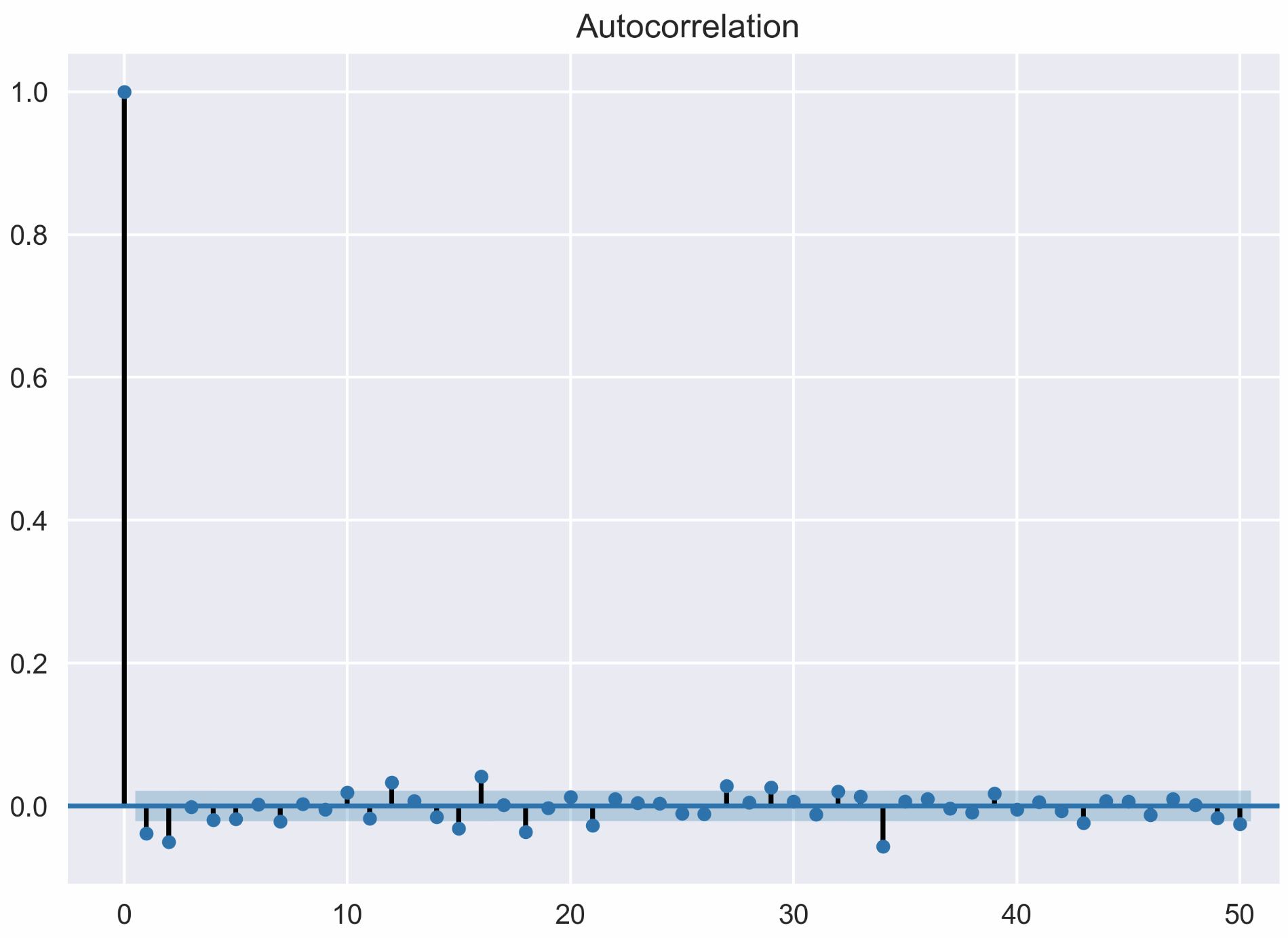Only a few values lie outside the confidence interval (we do not look at lag 0) and can be considered statistically significant. We can assume that we have verified that there is no autocorrelation in the log returns series. # Small and decreasing autocorrelation in squared/absolute returns Investigate this fourth fact by creating the ACF plots of squared and absolute returns. 1. Create the ACF plots: fig, ax = plt.subplots(2, 1, figsize=(12, 10))smt.graphics.plot_acf(df.log_rtn ** 2, lags=N_LAGS, alpha=SIGNIFICANCE_LEVEL, ax = ax)ax.set(title='Autocorrelation Plots', ylabel='Squared Returns')smt.graphics.plot_acf(np.abs(df.log_rtn), lags=N_LAGS, alpha=SIGNIFICANCE_LEVEL, ax = ax)ax.set(ylabel='Absolute Returns', xlabel='Lag') Executing the preceding code results in the following plots: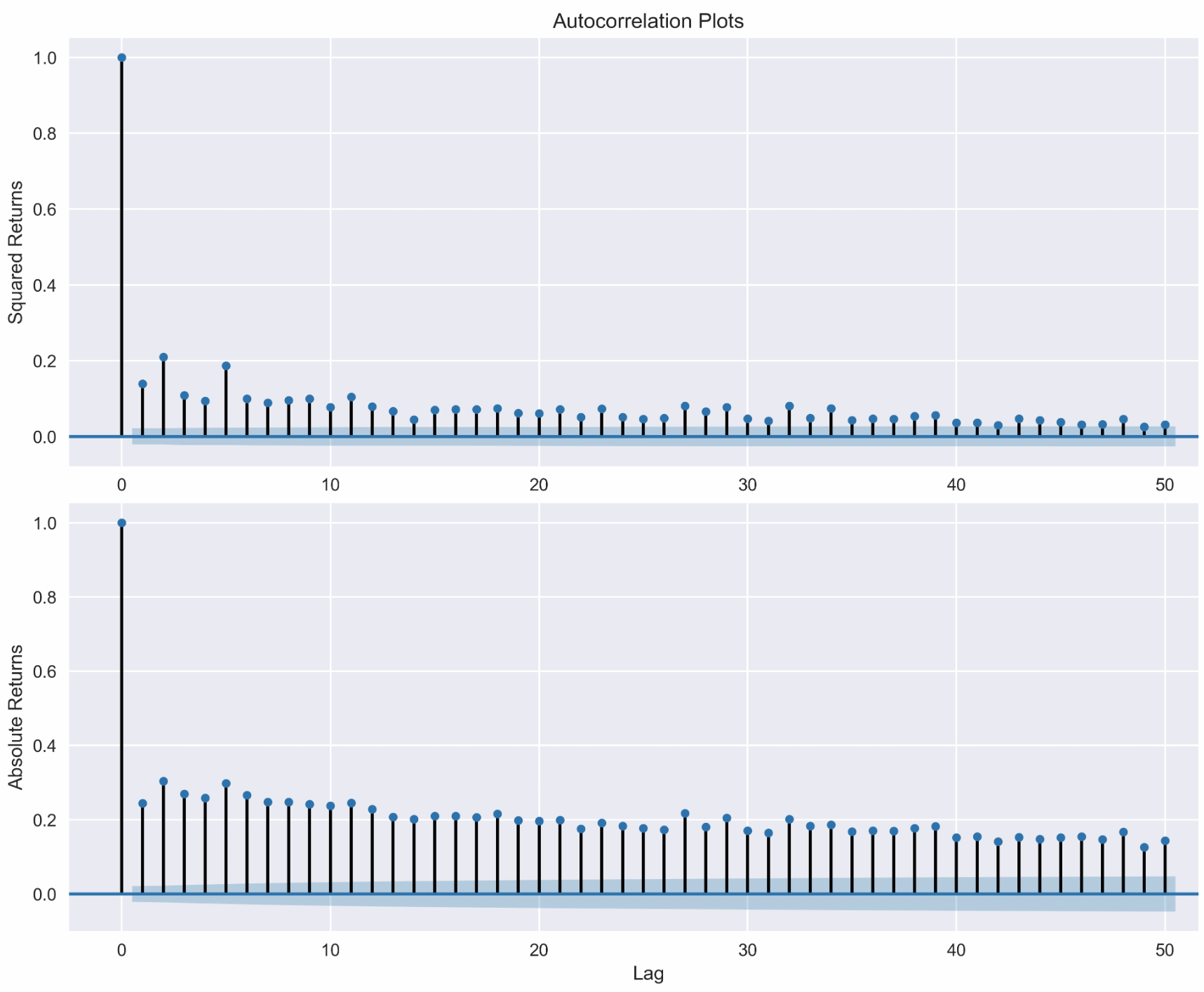We can observe the small and decreasing values of autocorrelation for the squared and absolute returns, which are in line with the fourth stylized fact. # Leverage effect For the fifth fact, run the following steps to investigate the existence of the leverage effect. 1. Calculate volatility measures as rolling standard deviations: df['moving_std_252'] = df[['log_rtn']].rolling(window=252).std()df['moving_std_21'] = df[['log_rtn']].rolling(window=21).std() 1. Plot all the series for comparison: fig, ax = plt.subplots(3, 1, figsize=(18, 15), sharex=True) df.adj_close.plot(ax=ax) ax.set(title='MSFT time series', ylabel='Stock price ($)')

df.log_rtn.plot(ax=ax)
ax.set(ylabel='Log returns (%)')

df.moving_std_252.plot(ax=ax, color='r',
label='Moving Volatility 252d')
df.moving_std_21.plot(ax=ax, color='g',
label='Moving Volatility 21d')
ax.set(ylabel='Moving Volatility',
xlabel='Date')
ax.legend()

We can now investigate the leverage effect by visually comparing the price series to the (rolling) volatility metric: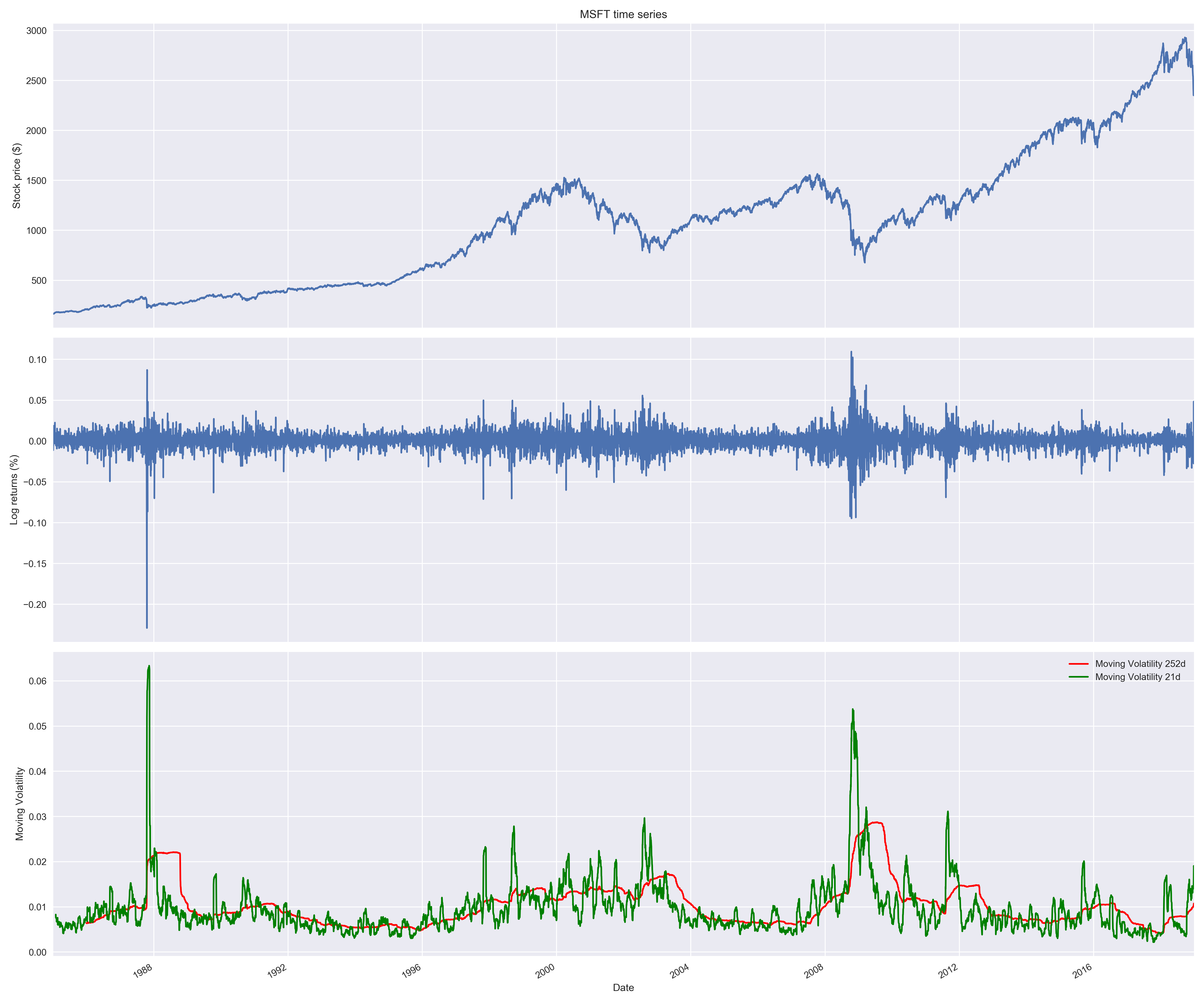This fact states that most measures of an asset's volatility are negatively correlated with its returns, and we can indeed observe a pattern of increased volatility when the prices go down and decreased volatility when they are rising.

# How it works...

In this section, we describe the approaches we used to investigate the existence of the stylized facts in the S&P 500 log return series.

# Fact 1

The name of the fact (Non-Gaussian distribution of returns) is pretty much self-explanatory. It was observed in the literature that (daily) asset returns exhibit the following:

• Negative skewness (third moment): Large negative returns occur more frequently than large positive ones.
• Excess kurtosis (fourth moment) : Large (and small) returns occur more often than expected.
The pandas implementation of kurtosis is the one that literature refers to as excess kurtosis or Fisher's kurtosis. Using this metric, the excess kurtosis of a Gaussian distribution is 0, while the standard kurtosis is 3. This is not to be confused with the name of the stylized fact's excess kurtosis, which simply means kurtosis higher than that of normal distribution.

We break down investigating this fact into three parts.

Histogram of returns

The first step of investigating this fact was to plot a histogram visualizing the distribution of returns. To do so, we used sns.distplot while setting kde=False (which does not use the Gaussian kernel density estimate) and norm_hist=True (this plot shows density instead of the count).

To see the difference between our histogram and Gaussian distribution, we superimposed a line representing the PDF of the Gaussian distribution with the mean and standard deviation coming from the considered return series.

First, we specified the range over which we calculated the PDF by using np.linspace (we set the number of points to 1,000, generally the more points the smoother the line) and then calculated the PDF using scs.norm.pdf. The default arguments correspond to the standard normal distribution, that is, with zero mean and unit variance. That is why we specified the loc and scale arguments as the sample mean and standard deviation, respectively.

To verify the existence of the previously mentioned patterns, we should look at the following:

• Negative skewness: The left tail of the distribution is longer, while the mass of the distribution is concentrated on the right side of the distribution.
• Excess kurtosis: Fat-tailed and peaked distribution.

The second point is easier to observe on our plot, as there is a clear peak over the PDF and we see more mass in the tails.

Q-Q plot

After inspecting the histogram, we looked at the Q-Q (quantile-quantile) plot, on which we compared two distributions (theoretical and observed) by plotting their quantiles against each other. In our case, the theoretical distribution is Gaussian (Normal) and the observed one comes from the S&P 500 returns.

To obtain the plot, we used the sm.qqplot function. If the empirical distribution is Normal, then the vast majority of the points will lie on the red line. However, we see that this is not the case, as points on the left side of the plot are more negative (that is, lower empirical quantiles are smaller) than expected in the case of the Gaussian distribution, as indicated by the line. This means that the left tail of the returns distribution is heavier than that of the Gaussian distribution. Analogical conclusions can be drawn about the right tail, which is heavier than under normality.

Descriptive statistics

The last part involves looking at some statistics. We calculated them using the appropriate methods of pandas Series/DataFrames. We immediately see that the returns exhibit negative skewness and excess kurtosis. We also ran the Jarque-Bera test (scs.jarque_bera) to verify that returns do not follow a Gaussian distribution. With a p-value of zero, we reject the null hypothesis that sample data has skewness and kurtosis matching those of a Gaussian distribution.

# Fact 2

The first thing we should be aware of when investigating stylized facts is the volatility clusteringperiods of high returns alternating with periods of low returns, suggesting that volatility is not constant. To quickly investigate this fact, we plot the returns using the plot method of a pandas DataFrame.

# Fact 3

Autocorrelation (also known as serial correlation) measures how similar is a given time series to the lagged version of itself, over successive time intervals.

To investigate whether there is significant autocorrelation in returns, we created the autocorrelation plot using plot_acf from the statsmodels library. We inspected 50 lags and used the default alpha=0.05, which means that we also plotted the 95% confidence interval. Values outside of this interval can be considered statistically significant.

# Fact 4

To verify this fact, we also used the plot_acf function from the statsmodels library; however, this time we applied it to the squared and absolute returns.

# Fact 5

This fact states that most measures of asset volatility are negatively correlated with their returns. To investigate it, we used the moving standard deviation (calculated using the rolling method of a pandas DataFrame) as a measure of historical volatility. We used windows of 21 and 252 days, which correspond to one month and one year of trading data.

# There's more...

We present another method of investigating the leverage effect (fact 5). To do so, we use the VIX (CBOE Volatility Index), which is a popular metric of the stock market's expectation regarding volatility. The measure is implied by option prices on the S&P 500 index. We take the following steps:

1. Download and preprocess the prices of the S&P 500 and VIX:
df = yf.download(['^GSPC', '^VIX'],                  start='1985-01-01',                  end='2018-12-31',                 progress=False)df = df[['Adj Close']]df.columns = df.columns.droplevel(0)df = df.rename(columns={'^GSPC': 'sp500', '^VIX': 'vix'})
1. Calculate the log returns (we can just as well use percentage change-simple returns):
df['log_rtn'] = np.log(df.sp500 / df.sp500.shift(1))df['vol_rtn'] = np.log(df.vix / df.vix.shift(1))df.dropna(how='any', axis=0, inplace=True)
1. Plot a scatterplot with the returns on the axes and fit a regression line to identify the trend:
corr_coeff = df.log_rtn.corr(df.vol_rtn)ax = sns.regplot(x='log_rtn', y='vol_rtn', data=df,                  line_kws={'color': 'red'})ax.set(title=f'S&P 500 vs. VIX ($\\rho$ = {corr_coeff:.2f})',       ylabel='VIX log returns',       xlabel='S&P 500 log returns')

We additionally calculated the correlation coefficient between the two series and included it in the title: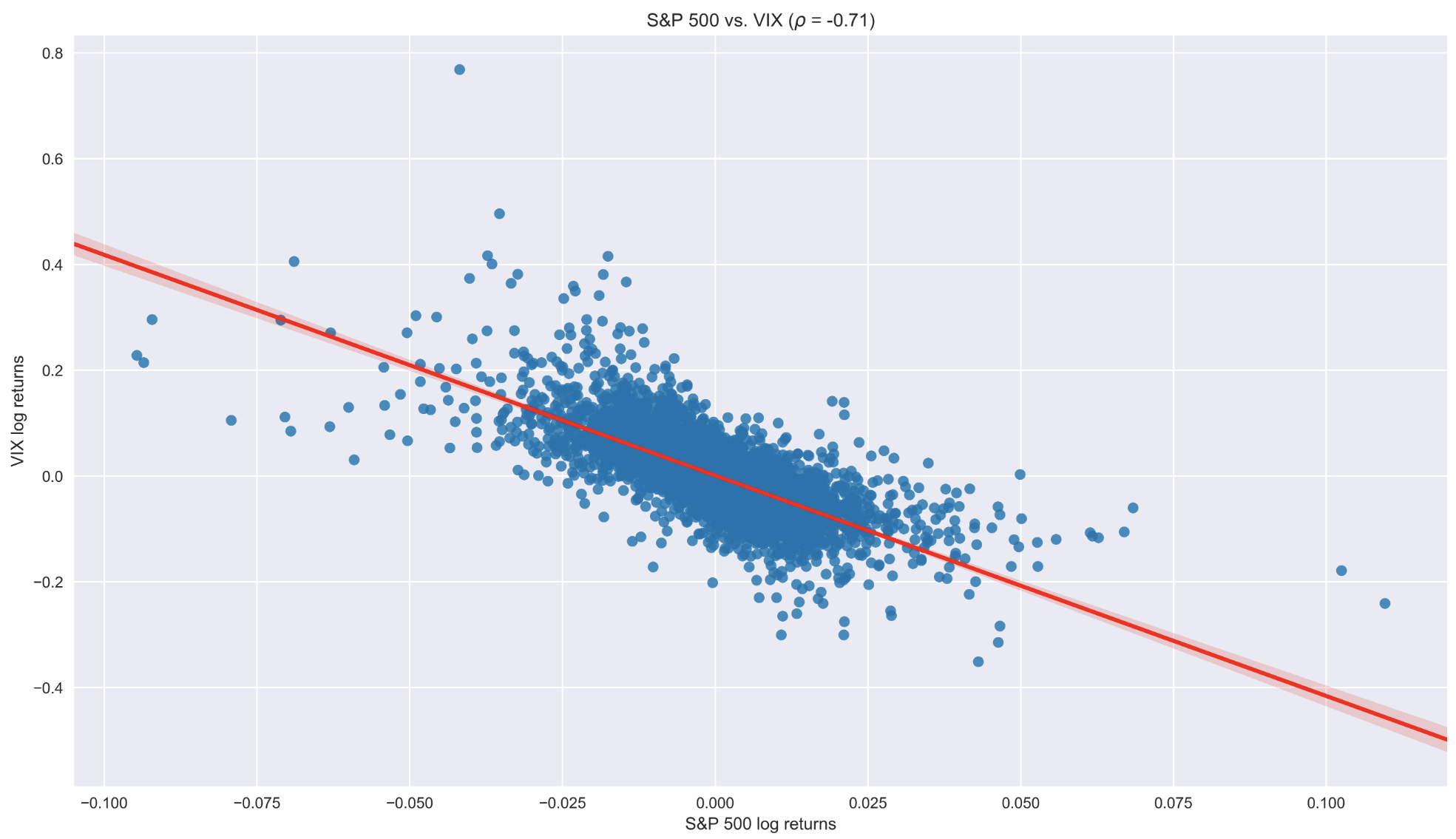We can see that both the negative slope of the regression line and a strong negative correlation between the two series confirm the existence of the leverage effect in the return series.

• Cont, R. (2001). Empirical properties of asset returns: stylized facts and statistical issues.